# Resistors in Parallel Formula

Resistors in Parallel Formula

In electric circuits, it is often possible to replace a group of resistors with a single, equivalent resistor. The equivalent resistance of a number of resistors in parallel can be found using the reciprocal of resistance, 1/R. The reciprocal of the equivalent resistance is equal to the sum of the reciprocals of each resistance. The unit of resistance is the Ohm (Ω), which is equal to a Volt per Ampere (1 Ω = 1 V/A). Larger resistors with kilo-Ohm (1 kΩ = 103 Ω) or mega-Ohm (1 MΩ = 106 Ω) resistances are common, as well.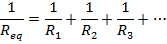Req = equivalent resistance (Ω or larger units)

R1 = resistance of first resistor (Ω)

R2 = resistance of second resistor (Ω)

R3 = resistance of third resistor (Ω)

Resistors in Parallel Formula Questions:

1) What is the equivalent resistance of a 1000 kΩ and a 250.0 kΩ resistor connected in parallel?

Answer: The resistances are both expressed in kilo-Ohms, and so there is no need to change their units. The equivalent resistance can be found in kΩ using the formula: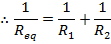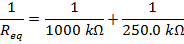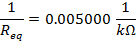The final step is to invert the values on both sides of the formula to find the equivalent resistance: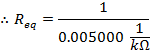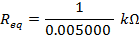Req = 200.0 kΩ

The equivalent resistance of the 1000 kΩ and 250.0 kΩ resistors in parallel is 200.0 kΩ.

2) Three resistors are connected in parallel in an electric circuit. Their resistances are 400 Ω, 40.0 kΩ, and 4.00 MΩ. What is the equivalent resistance?

Answer: The three resistance values are expressed using different units. The first step to finding the equivalent resistance is to convert these to a common unit. Two of the values can be converted to the same unit as the third. In this solution, all of the values will be converted to Ohms.

If R1 = 400 Ω, R2 = 40.0 kΩ, and R3 = 4.00 MΩ, then:

R2 = 40.0 kΩR2 = 40 000 Ω

The value of R3 is:

R3 = 4.00 MΩ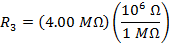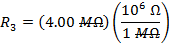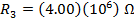R3 = 4 000 000 Ω

The equivalent resistance can be found in Ohms using the formula: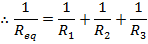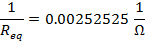The final step is to invert the values on both sides of the formula to find the equivalent resistance: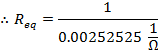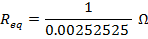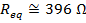The equivalent resistance of the 400 Ω, 40.0 kΩ, and 4.00 MΩ resistors in parallel is approximately 396 Ω.